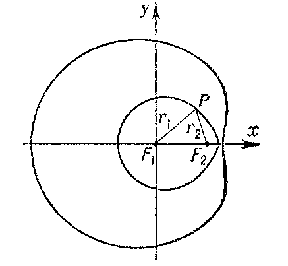# Descartes oval

(diff) ← Older revision | Latest revision (diff) | Newer revision → (diff)

A plane curve for which the distances $r_1$ and $r_2$ between any point $P$ of the curve and two fixed points $F_1$ and $F_2$ (the foci) are related by the non-homogeneous linear equation

$$r_1+mr_2=a.$$

A Descartes oval may be defined by means of the homogeneous linear equation

$$r_1+mr_2+nr_3=0,$$

where $r_3$ is the distance to the third focus $F_3$ located on the straight line $F_1F_2$. In the general case, a Descartes oval consists of two closed curves, one enclosing the other (see Fig.). The equation of a Descartes oval in Cartesian coordinates is

$$\sqrt{x^2+y^2}+m\sqrt{(x-d)^2+y^2}=a,$$

where $d$ is the length of the segment $F_1F_2$. If $m=1$ and $a>d$, the Descartes oval is an ellipse; if $m=-1$ and $a<d$, it is a hyperbola; if $m=a/d$, it is a Pascal limaçon. First studied by R. Descartes in the context of problems of optics .Figure: d031340a

There are several ways in which a Cartesian oval can arise. One is as follows. Let $C$ and $C_1$ be two cones with axes parallel to the $z$-axis and with circular intersections with the $xy$-plane. Then the vertical projection onto the $xy$-plane of their intersection is a Cartesian oval. This is a result of A. Quetelet. Suppose the apexes of $C$ and $C_1$ are at $(0,0,1)$ and $(c,0,a)$, respectively, and the intersections with the $xy$-plane are circles of radius 1 and $b$ (see below). Then in the case $b=(1-a)^{-1}a(a+1)$, $c=-(1-a)^{-1}(a+1)^2$ the projection of the intersection is a cardioid.
In polar coordinates the equation for a Cartesian oval is $r^2-2(a+b\cos\phi)r+c^2=0$. Hence when $c=0$ the equation becomes $r=a+b\cos\phi$ (plus $r=0$) and one obtains the Pascal limaçon; when in addition $a=b$, the cardioid results, whose equation can also be written as $\sqrt r=\sqrt m\cos(\phi/2)$.